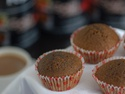## Ratios

Type of Unit: Concept

# Prior Knowledge

Students should be able to:

• Calculate with whole numbers up to 100 using all four operations.
• Understand fraction notation and percents and translate among fractions, decimal numbers, and percents.
• Interpret and use a number line.
• Use tables to solve problems.
• Use tape diagrams to solve problems.
• Sketch and interpret graphs.
• Write and interpret equations.

# Lesson Flow

The first part of the unit begins with an exploration activity that focuses on a ratio as a way to compare the amount of egg and the amount of flour in a mixture. The context motivates a specific understanding of the use of, and need for, ratios as a way of making comparisons between quantities. Following this lesson, the usefulness of ratios in comparing quantities is developed in more detail, including a contrast to using subtraction to find differences. Students learn to interpret and express ratios as fractions, as decimal numbers, in a:b form, in words, and as data; they also learn to identify equivalent ratios.

The focus of the middle part of the unit is on the tools used to represent ratio relationships and on simplifying and comparing ratios. Students learn to use tape diagrams first, then double number lines, and finally ratio tables and graphs. As these tools are introduced, students use them in problem-solving contexts to solve ratio problems, including an investigation of glide ratios. Students are asked to make connections and distinctions among these forms of representation throughout these lessons. Students also choose a ratio project in this part of the unit (Lesson 8).

The third and last part of the unit covers understanding percents, including those greater than 100%.

Students have ample opportunities to check, deepen, and apply their understanding of ratios, including percents, with the selection of problems in the Gallery.

Subject:
Mathematics, Statistics and Probability
Material Type:
Unit of Study
Level:
Middle School
6
Tags:
Comparison, Cooking, Ingredients, Percentages, Equivalence, Whole-Part Statements, Multiplicative Structure, Value, Graphs, 6th Grade Mathematics, Mixtures, Values, Quantity, Equivalent Ratios, Double Number Line, Glide Ratios, Percent Statements, Proportions, Tape Diagrams, Double Number Lines, Percent Problems, Constant Ratio, Numbers, Ratios, Problem-Solving, Ratio Tables
Unit 3 Ratios•••••••••••Lesson 11

Glide Ratio
••••••••Lesson 19

Peer Review